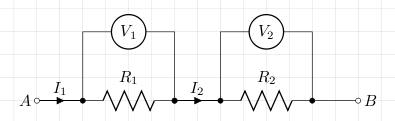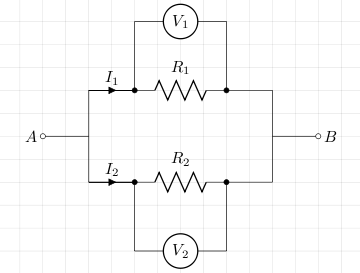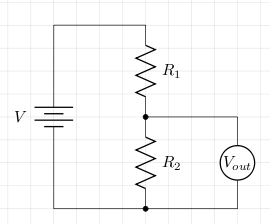# Resistor circuit (series, parallel, voltage divider)

In circuits resistances (resistors) are often found combined in series or parallel. In the following it is shown how to calculate total resistance in such cases.

## Series

In the case of resistors in series, the same current flows through each resistor. The voltage across the resistors is the sum of the voltages across each individual resistor. The total resistance is the sum of all resistances.```RAB = R1 + R2
VAB = V1 + V2
IAB = I1 = I2
```

## Parallel

In the case of resistors in parallel, the current splits but all resistors have the same voltage across them. The total resistance is calculated from the reciprocal of the resistances (shown below).```VAB = V1 = V2
IAB = I1 + I2

IAB = VAB/R1 + VAB/R2 = VAB(1/R1 + 1/R2)
IAB/VAB = 1/R1 + 1/R2
so
1/RAB = 1/R1 + 1/R2, or
RAB = R1 × R2/(R1 + R2)
```

## Voltage Divider

A voltage divider is built from two resistors in series. The voltage across an individual resistor is a fraction of the voltage across both. This fraction is the ratio of the resistance of the individual resistor to the total resistance of both resistors.```V = I(R1 + R2)
Vout = IR2
Vout/V = R2/(R1 + R2)
```

## References

Fischer-Cripps. A.C., The Electronics Companion. Institute of Physics, 2005.